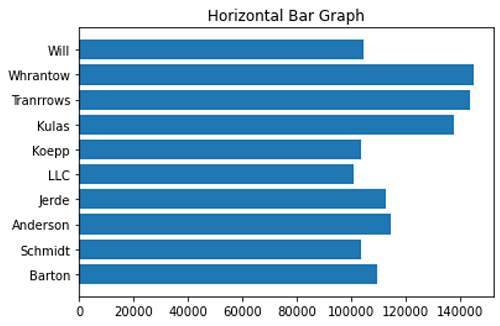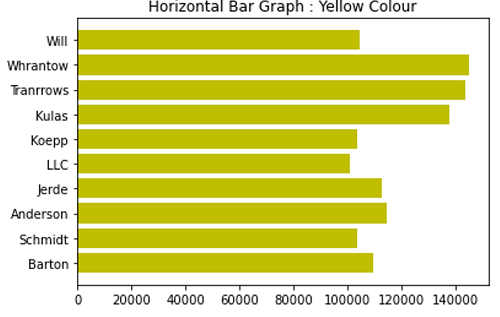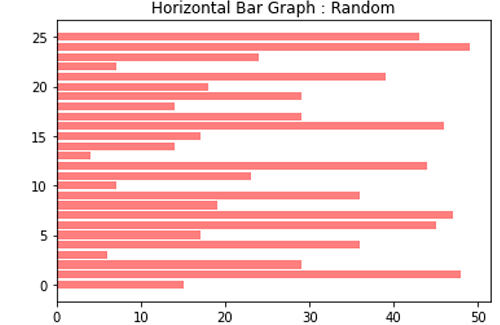# Python | Horizontal Bar Graph

Python | Horizontal Bar Graph: In this article, we are going to learn about the horizontal bar graph and its Python implementation.
Submitted by Anuj Singh, on July 13, 2020

A bar graph is a type of data visualization technique that is very often used to represent data in the form of a vertical bar and some cases of horizontal bars. The length of the bar is proportional to the value. They allow us to compare data very clearly and therefore, bar graphs or bar charts are included in most of the presentations worldwide. A bar graph can be used to show comparisons between discrete classes and one of the two axes represents the specific categories that are being compared, and the other axis represents a measured value.

matplotlib allows us to use this particular class of data visualization via an inbuilt function matplotlib.barh().

The following are examples for understanding the implementation of bar graphs.

## 1) Standard Bar Graph

Syntax:

```plt.barh(x, y)
```
• x - names/numeric distribution
• y - length of the bar## 2) Horizontal Bar Graph with Color Change (Yellow)

Syntax:

```plt.barh(x, y, color='y')
```
• x - names/numeric distribution
• y - length of the bar
• color='g' - yellow color bars## 3) A Random Horizontal Bar graph with using opaque ratio

Syntax:

```plt.barh(
np.arange(26),
np.random.randint(0,50,26),
alpha = 0.5,
color='r')
```
• alpha=0.6 - opaque ratio as 0.6## Python code for horizontal bar graph

```# Data Visualisation using Python
# Horizontal Plotting

import numpy as np
import matplotlib.pyplot as plt
from matplotlib.ticker import FuncFormatter

data = {'Barton': 109448.50,
'Schmidt': 103469.59,
'Anderson': 114214.71,
'Jerde': 112541.43,
'LLC': 100944.30,
'Koepp': 103460.54,
'Kulas': 137451.96,
'Tranrrows': 143381.38,
'Whrantow': 144841.99,
'Will': 104447.60}

group_data = list(data.values())
group_names = list(data.keys())

# Default Settings
plt.figure()
plt.barh(group_names, group_data)
plt.title('Horizontal Bar Graph')

# Yellow Colour
plt.figure()
plt.barh(group_names, group_data, color='y')
plt.title('Horizontal Bar Graph : Yellow Colour')

# Random Bar Graph Example
plt.figure()
plt.barh(np.arange(26), np.random.randint(0,50,26), alpha = 0.5, color='r')
plt.title('Horizontal Bar Graph : Random')
```

Output:

```Output is as figure
```

Languages: » C » C++ » C++ STL » Java » Data Structure » C#.Net » Android » Kotlin » SQL
Web Technologies: » PHP » Python » JavaScript » CSS » Ajax » Node.js » Web programming/HTML
Solved programs: » C » C++ » DS » Java » C#
Aptitude que. & ans.: » C » C++ » Java » DBMS
Interview que. & ans.: » C » Embedded C » Java » SEO » HR
CS Subjects: » CS Basics » O.S. » Networks » DBMS » Embedded Systems » Cloud Computing
» Machine learning » CS Organizations » Linux » DOS
More: » Articles » Puzzles » News/Updates